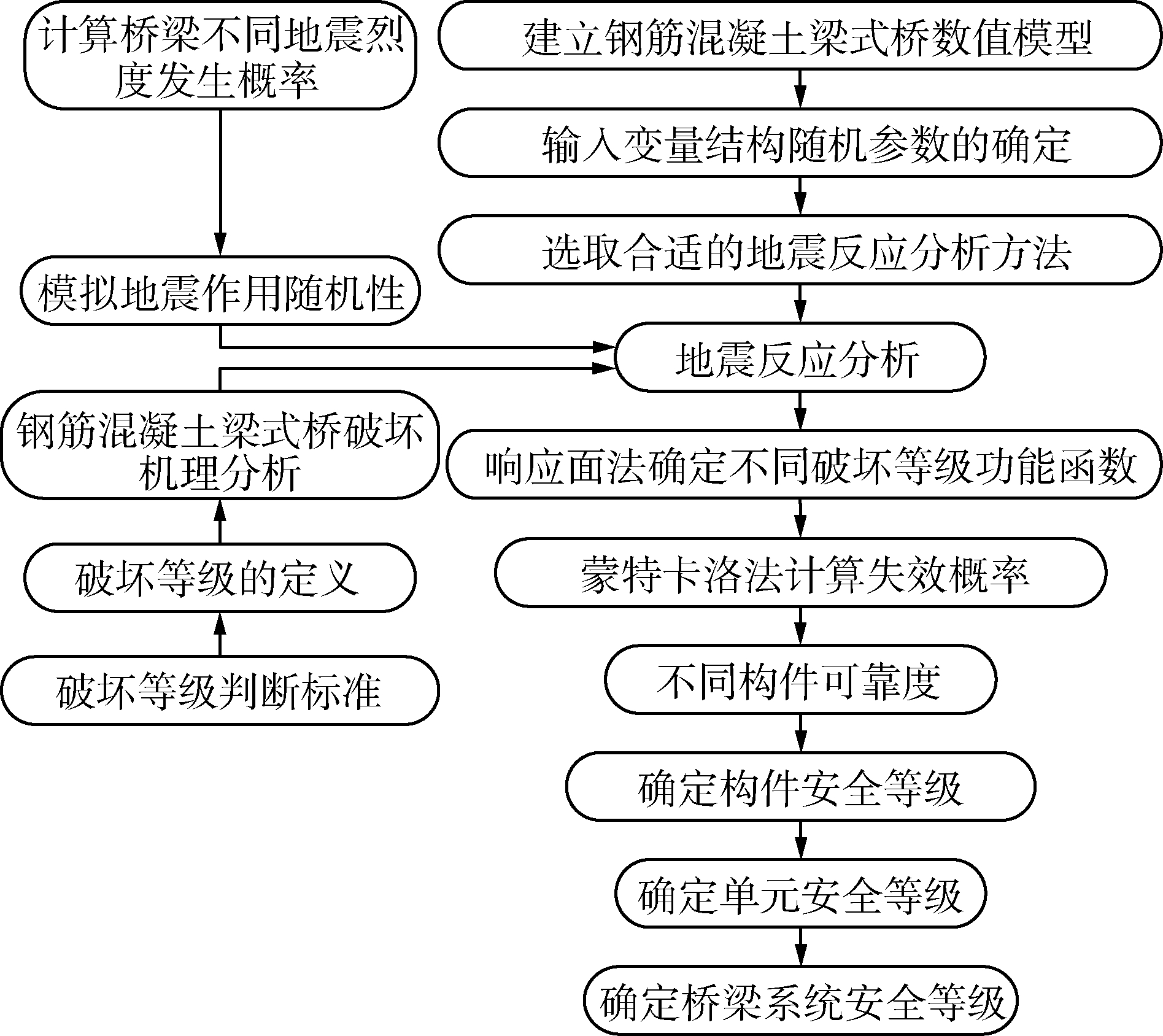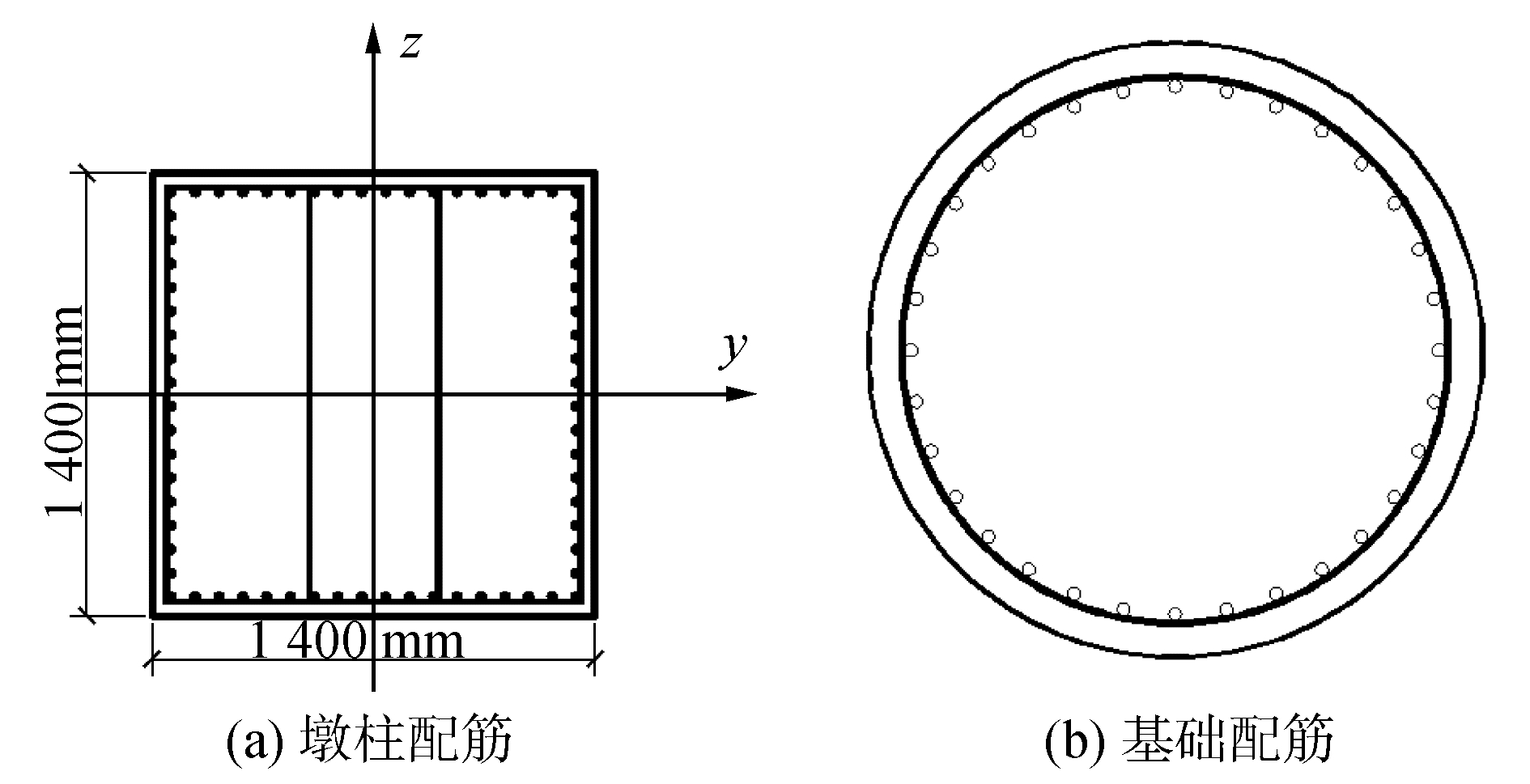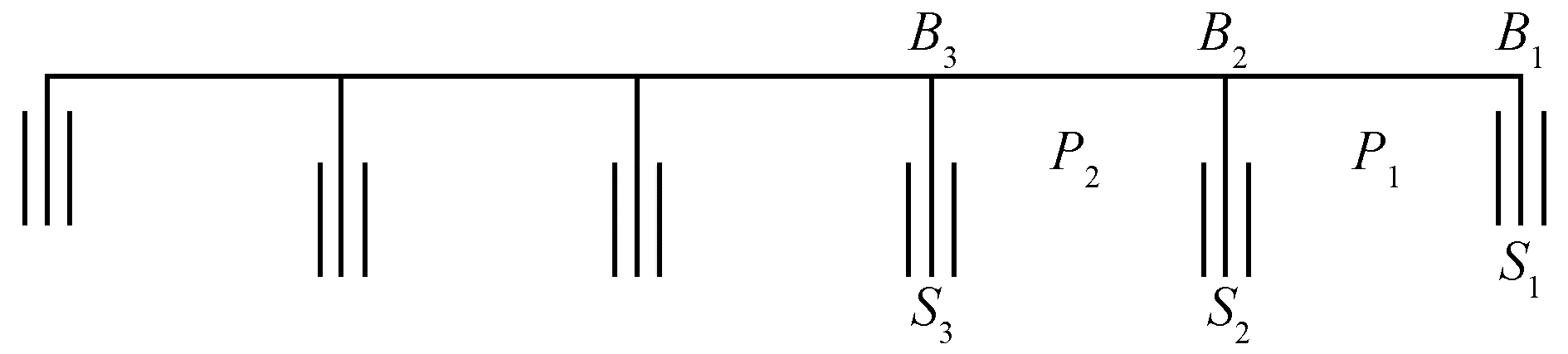﻿ 钢筋混凝土梁式桥地震安全性分析及评价
«上一篇文章快速检索 高级检索

 哈尔滨工程大学学报2019, Vol. 40Issue (4): 710-717  DOI: 10.11990/jheu.2017090750

引用本文ZHAO Jun, LIN Junqi, LIU Jinlong, et al. Analysis and evaluation on earthquake safety evaluation of RC beam bridges[J]. Journal of Harbin Engineering University, 2019, 40(4), 710-717. DOI: 10.11990/jheu.201709075.文章历史

1. 中国地震局工程力学研究所, 地震工程与工程振动重点实验室, 黑龙江 哈尔滨 150080;
2. 哈尔滨工程大学 航天与建筑工程学院, 黑龙江 哈尔滨 150001

Analysis and evaluation on earthquake safety evaluation of RC beam bridges
ZHAO Jun 1, LIN Junqi 1, LIU Jinlong 1, WANG Baolai 2
1. Key Laboratory of Earthquake Engineering and Engineering Vibration, China Earthquake Administration, Institute of Engineering Mechanics, China Earthquake Administration, Harbin 150080, China;
2. College of Aerospace and Civil Engineering, Harbin Engineering University, Harbin 150080, China
Abstract: To solve the earthquake safety problems of bridges, this study analyzed the bridge failure mechanism based on reliability theory by considering the randomness of material strength and uncertainties of computing models, which have important effects on structural resistance force.Failure probabilities of RC beam bridge piers, bearings, upper structures, and pier foundations under frequent, occasional, and rare earthquake levels, as well as seismic reliabilities of bridge members in the basic design period, were calculated by the response surface analysis method.In addition, different seismic safety grades of the bridge and its components were given to evaluate the earthquake safety level of the bridge.An RC beam bridge was modeled and its safety grades were given.The conclusion illustrates that the RC beam bridge has a high safety level under earthquake in the design period.
Keywords: reliability theory    reinforced concrete continuous girder bridge    seismic safety    safety evaluation index    function    response surface method    component    bridge system

1 桥梁的破坏机理

2 地震可靠度理论

2.1 集成化响应面法

 $\hat T\left( x \right) = a + \sum\limits_{i = 1}^n {{b_i}{x_i}} + \sum\limits_{i = 1}^n {{c_i}x_i^2}$ (1)

 $\hat T\left( x \right) = a + \sum\limits_{i = 1}^n {{b_i}{x_i}} + \sum\limits_{i = 1}^n {{c_i}x_i^2} + \sum\limits_{i = 1}^n {\sum\limits_{j < i}^n {{d_{ij}}{x_i}{x_j}} }$ (2)

2.2 破坏等级的划分及功能函数

 $\begin{array}{*{20}{c}} {\left\{ {{B_1},{B_2},{B_3}} \right\} = \left\{ {无损伤,轻微或中等损伤,} \right.}\\ {\left. {严重损伤或破坏} \right\}} \end{array}$ (3)

B1：结构或构件的强度不超过弹性极限、变形小于弹性允许变形，形成非结构损伤；

B2：结构或构件的强度超过弹性极限或变形大于弹性允许变形，但变形小于弹塑性允许变形，形成结构性损伤；

B3：结构或构件变形大于弹塑性允许变形，具有结构性损伤。

2.3.1 损伤初形成极限状态

 ${Z_{D1}} = {M_{yD}} - {M_s}$ (4)
 ${Z_{D2}} = {\mu _1} - {\mu _z}$ (5)
 ${Z_{D3}} = {M_{c1}} - {M_{s2}}$ (6)

 ${\mu _z} = {u_{\max }}/{X_E}$ (7)

2.3.2 损伤形成后极限状态

 ${Z_1} = {M_u} - {M_s}$ (8)
 ${Z_2} = {V_n} - V$ (9)
 ${Z_3} = {\mu _2} - {\mu _d}$ (10)

 ${\mu _d} = \Delta /{\Delta _{cy1}}$ (11)

 ${Z_4} = {\mu _3} - {\mu _z}$ (12)
 ${Z_5} = {M_y} - {M_{s2}}$ (13)
 ${Z_6} = {V_{c2}} - {V_{s2}}$ (14)
 ${Z_7} = {P_0} - {P_H}$ (15)

 ${P_0} = \frac{1}{2}u\sum\limits_{i = 1}^n {{q_{ik}}{l_i}} + {A_p}{m_0}\lambda \left\{ {\left[ {{f_{a0}}} \right] + {k_2}{\gamma _2}\left( {h - 3} \right)} \right\}$ (16)表 1 桥墩、支座和桩基础由功能函数定义的损伤状态 Table 1 Damage states determined by functions of piers and bearings

 $\begin{array}{*{20}{c}} {{Z_{D1}} = {\alpha _{D1}}{{\hat T}_{D1}}\left( {{x_1},{x_2}, \cdots ,{x_n}} \right)}\\ {{Z_{D2}} = {\alpha _{D2}}{{\hat T}_{D2}}\left( {{x_1},{x_2}, \cdots ,{x_n}} \right)}\\ {{Z_{D3}} = {\alpha _{D3}}{{\hat T}_{D3}}\left( {{x_1},{x_2}, \cdots ,{x_n}} \right)} \end{array}$ (17)

 $\begin{array}{*{20}{c}} {{Z_1} = {\alpha _1}{{\hat T}_1}\left( {{x_1},{x_2}, \cdots ,{x_n}} \right)}\\ {{Z_2} = {\alpha _2}{{\hat T}_2}\left( {{x_1},{x_2}, \cdots ,{x_n}} \right)}\\ {{Z_3} = {\alpha _3}{{\hat T}_3}\left( {{x_1},{x_2}, \cdots ,{x_n}} \right)}\\ {{Z_4} = {\alpha _4}{{\hat T}_4}\left( {{x_1},{x_2}, \cdots ,{x_n}} \right)}\\ {{Z_5} = {\alpha _5}{{\hat T}_5}\left( {{x_1},{x_2}, \cdots ,{x_n}} \right)}\\ {{Z_6} = {\alpha _6}{{\hat T}_6}\left( {{x_1},{x_2}, \cdots ,{x_n}} \right)}\\ {{Z_7} = {\alpha _7}{{\hat T}_7}\left( {{x_1},{x_2}, \cdots ,{x_n}} \right)} \end{array}$ (18)
2.4 地震可靠度

 $\begin{array}{l} {P_f}\left( {{B_i}} \right) = P\left( {{I_s}} \right){P_{{I_s}}}\left( {{B_i}} \right) + P\left( {{I_o}} \right){P_{{I_o}}}\left( {{B_i}} \right) + \\ \;\;\;\;\;\;\;\;\;\;\;\;\;\;P\left( {{I_l}} \right){P_{{I_l}}}\left( {{B_i}} \right) \end{array}$ (19)

 $\begin{array}{*{20}{c}} {P\left( {{I_s}} \right) = {P_l}\left( {{I_o} - 0.77} \right)}\\ {P\left( {{I_o}} \right) = {P_l}\left( {{I_o} + 0.5} \right) - {P_l}\left( {{I_o} - 0.77} \right)}\\ {P\left( {{I_l}} \right) = 1 - {P_l}\left( {{I_o} + 0.5} \right)} \end{array}$ (20)

 ${P_s} = 1 - {P_f} = 1 - \int_0^\infty {{P_f}\left( {R < S\left| a \right.} \right)f\left( a \right){\rm{d}}a}$ (21)

3 钢筋混凝土梁式桥的地震安全性

3.1 安全评价指标

 $S = \sum\limits_{i = 1}^3 {{P_s}\left( {{B_i}} \right){K_{{B_i}}}}$ (22)表 2 桥梁构件的安全水平划分 Table 2 Safety level division of bridge components
3.2 桥梁单元及系统的地震安全性等级划分表 3 桥梁单元的安全等级划分 Table 3 Safety grade division of the bridge units表 4 桥梁系统的安全等级划分 Table 4 Safety grade division of the bridge structure
3.3 计算步骤

1) 根据式(20)计算地震烈度IsIoIl在设计基准期内发生的概率P(Is)、P(Io)和P(Il)。

2) 确定需要输入的随机变量和输出变量。本文采用表 5中的输入变量，并由拉丁超立方抽样法(LHS)获得。输出变量根据不同功能函数的表现形式，一般取为结构或构件在地震激励下的响应，本文为桥墩的弯矩、剪力、相对位移延性比、支座的相对位移延性比、基础的弯矩、剪力和轴力。输出变量可以按照反应谱法或其他动力方法进行FEM分析得到。本文算例采用规范反应谱法进行确定性分析。表 5 随机变量统计特征 Table 5 Statistics of random variables

3) 根据桥墩、支座和基础的功能函数(4)~(6)、(8)~(14)，运用RSM法构造不同地震烈度IsIoIl下的响应面函数$\hat T$(x)(式(1)或式(2))，用回归分析方法和Matlab程序结合可靠度方法中的JC法求得函数中的待定系数，最终得到式(17)和(18)。

4) 根据响应面分析得到的近似函数$\hat T$(x)和满足失效概率精度要求的数值模拟次数，利用Matlab程序在响应面上生成MCS样本，根据功能函数(17)和(18)计算桥墩、支座和基础在不同地震烈度下不同损伤状态的失效概率。

5) 得到PIs(Bi)、PIo(Bi)、PIl(Bi)后，按式(19)计算构件设计基准期内发生3个破坏等级的失效概率，然后用式(21)求出构件的可靠度。

6) 用安全评价指标计算式(22)将构件的可靠度转化为安全评价指标，评价构件的安全等级。根据表 34评价桥梁单元和系统的安全等级。Download: 图 1 钢筋混凝土梁式桥地震安全性评价模型 Fig. 1 Model of Seismic safety evalution of the reinforced concrete beam bridge
4 钢筋混凝土梁式桥计算算例Download: 图 2 墩柱及基础配筋 Fig. 2 Pier and foundation reinforcementDownload: 图 3 钢筋混凝土连续梁桥有限元模型 Fig. 3 Finite element model of the reinforced concrete continuous beam bridge表 6 地震烈度的发生概率 Table 6 The probabilities of seismic intensities表 7 桥墩在不同地震烈度下不同破坏等级的失效概率 Table 7 The failure probability of piers under different seismic intensities表 8 支座在不同地震烈度下不同破坏等级的失效概率 Table 8 The failure probability of bearings under different seismic intensities表 9 基础在不同地震烈度下不同破坏等级的失效概率 Table 9 The failure probability of foundations under different seismic intensities表 10 桥墩在设计基准期内发生不同种损伤情况可靠度 Table 10 The reliability of different damages of piers in the design period表 11 支座在设计基准期内发生不同种损伤情况可靠度 Table 11 The reliability of different damages of bearings in the design period表 12 基础在设计基准期内发生不同破坏等级的可靠度 Table 12 The reliability of different damages of foundations in the design period表 13 构件的安全性评价指标 Table 13 Safety evaluation index of components表 14 桥梁单元和系统的安全等级 Table 14 Safety grades of the bridge structure and its components

5 结论

1) 以可靠度理论作为出发点，定义了地震安全评价指标和桥梁单元，以此评价桥梁的地震安全性，判断其损伤程度、安全等级和履行功能情况。通过算例，从理论上验证了改进该理论后评价桥梁地震安全性的可行性。

2) 以往应用可靠度理论只是给出了构件或结构发生完全破坏的可能性，本文结合破坏准则，采用不同损伤极限状态下的功能函数，可以分析出构件不同破坏等级发生的可能性，并给出相应的安全水平和功能，为桥梁的加固维修、抗震救灾等提供了参考。

  姜淑珍, 林均岐. 城市桥梁震害预测方法的探讨[J]. 世界地震工程, 2004, 20(4): 64-66. JIANG Shuzhen, LIN Junqi. Study on seismic damage predicting methods of bridges in cities[J]. World information on earthquake engineering, 2004, 20(4): 64-66. DOI:10.3969/j.issn.1007-6069.2004.04.011 (0)  王东升, 冯启民. 桥梁震害预测方法[J]. 自然灾害学报, 2001(3): 113-118. WANG Dongsheng, FENG Qimin. Seismic damage assessment methods for bridges[J]. Journal of natural disasters, 2001(3): 113-118. DOI:10.3969/j.issn.1004-4574.2001.03.020 (0)  CHOI E, DESROCHES R, NIELSON B. Seismic fragility of typical bridges in moderate seismic zones[J]. KSCE journal of civil engineering, 2003, 7(1): 41-51. DOI:10.1007/BF02841989 (0)  陈亮. 钢筋混凝土桥梁地震易损性分析方法[J]. 上海公路, 2007, 3: 48-51. CHEN Liang. Seismic fragility analytical methodology for reinforced concrete bridges[J]. Shanghai Highways, 2007, 3: 48-51. (0)  王占飞, 张济宁, 赵乃志. 日本高速道路桥梁抗震设计方法的研究[J]. 世界桥梁, 2008, 4: 13-15. WANG Zhanfei, ZHANG Jining, ZHAO Naizhi. Study of seismic design methods for expressway bridges in japan[J]. World Bridges, 2008, 4: 13-15. (0)  武清玺. 结构可靠度理论、方法及应用[M]. 北京: 科学出版社, 2004: 39-84. WU Qingxi. Structural reliability theory, method and application[M]. Beijing: Science Press, 2004: 39-84. (0)  叶爱君. 桥梁抗震[M]. 北京: 人民交通出版社, 2011: 14-32. YE Aijun. Seismic design of bridges[M]. Beijing: China Communications Press, 2011: 14-32. (0)  陈文元, 赵雷, 钟强文, 等. 基于响应面法的连续刚构地震可靠度分析[J]. 地震工程与工程振动, 2009, 29(3): 45-50. CHEN Wenyuan, ZHAO Lei, ZHONG Qiangwen, et al. Seismic reliability analysis of rigid frame bridge based on response surface method[J]. Journal of Earthquake Engineering & Engineering Vibration, 2009, 29(3): 45-50. (0)  程进. 基于响应面法的几何非线性结构概率响应分析[J]. 同济大学学报(自然科学版), 2006, 34(9): 1147-1151. CHENG Jin. Probabilistic response analysis of geometrically nonlinear structures based on systematic response surface method[J]. Journal of Tongji University(Natural science), 2006, 34(9): 1147-1151. DOI:10.3321/j.issn:0253-374X.2006.09.003 (0)  欧进萍, 段宇博. 高层建筑结构的抗震可靠度分析与优化设计[J]. 地震工程与工程振动, 1995, 15(1): 1-13. OU Jinping, DUAN Yubo. Seismic reliability analysis and optimum design of tall buildings[J]. Earthquake Engineering & Engineering Vibration, 1995, 15(1): 1-13. (0)  Motoyuki Suzuki, Mitsuyoshi Akiyama, Yasunori Yamazaki, et al. Method of evaluating earthquake safety of RC bridge system based on reliability theory[J]. Concrete Library International of JSCE, 2001, 38: 33-50. (0)  JTC/TB02-01-2008.公路桥梁抗震设计细则[S].北京: 人民交通出版社, 2008. JTC/TB02-01-2008. Guidelines for seismic design of highway bridges[S]. Beijing: China Communications Press, 2008. (0)  谢旭. 桥梁结构地震响应分析与抗震设计[M]. 北京: 人民交通出版社, 2006: 311-313. XIE Xu. Seismic response analysis and seismic design of bridge structures[M]. Beijing: China Communications Press, 2006: 311-313. (0)  ASSOCIATION J R. Specifications forhighway bridges, Part Ⅳ substructures[M]. Japan: Maruzen, 1996. (0)  SUZUKI M, AKIYAMA M, YAMAZAKI Y. Safety evaluation method of structural system and its application to seismic design of RC bridge pier[J]. Concrete library international of JSCE, 1997, 32: 43-68. (0)  李立峰, 吴文朋, 黄佳梅, 等. 地震作用下中等跨径RC连续梁桥系统易损性研究[J]. 土木工程学报, 2012, 45(10): 152-160. LI Lifeng, WU Wenpeng, HUANG Jiamei, et al. Study on system vulnerability of medium span reinforced concrete continuous girder bridge under earthquake excitation[J]. China Civil Engineering Journal, 2012, 45(10): 152-160. (0)  ZHAO Jun, LIN Junqi, LIU Jinlong, et al. Study on safety level of RC beam bridges under earthquake[C]//ACNCIS, 2017, Zhengzhou: AIP Comference Proceedings. (0)